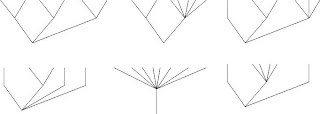## M Theory Lesson 73

Twistor space is 6 dimensional (over the reals). If we ask ourselves which 2-level polytopes might be used to model such a space we find that there are quite a few, namely those labelled by the trees shown, along with obvious permutations and variants on the (2,5) leaved case.The 8 leaved rooted tree will be familiar as the Stasheff associahedron sitting in \$\mathbb{R}^6\$. The first 6 leaved example is a typical element of the notorious counterexamples discovered by Tamarkin, and presumably plays a role in describing the 6 dimensional moduli \$M_{2,0}\$ and \$M_{0,6}\$ which feature so prominently in M Theory.

## 4 Responses so far »

1. 1### nige said,

Ummm. Are you now starting to discuss the other M-theory, that with 6 spatial dimensions in the Calabi-Yau manifold?

The tree structure diagrams are always interesting to me, since nodes (or triangular vertices) where the lines meet, can also be used to represent charges (i.e., masses are gravitational charges) distributed throughout the universe in space. Let the lines represent the paths of gauge boson radiation, and the vertices represent masses (i.e. higgs quanta, or whatever). All you need to do to calculate gravity is to count up the lines like little arrows. The result is a simplified path integral.

According to Feynman, gauge bosons should travel in straight lines unless they are deflected by interactions with virtual fermions (which generally only arise in strong fields, above the energy for pair production). Even when they are deflected, if gravity is quantized the deflection should be quantized (i.e., the path should be two straight lines meeting at point where the graviton interacted with the photon via a higgs boson or whatever).

So there should be a mathematical way of describing path integrals by tree diagrams with straight lines at low energy (below the pair production threshold).

The problem is of course that light gets deflected by gravity, as shown by the 1919 ellipse. If quantum gravity is correct, the photon is deflected in discrete steps by gravitons, not by a smooth spacetime continuum (i.e., a jagged line composed of lots of little straight lines should replace the smooth curvature of the line given by the Ricci tensor).

What’s fascinating about the exchange radiation model for forces is the effect of accelerations, and motion generally.

When two particles move apart, which are exchanging gauge bosons, won’t the gauge bosons be redshifted, losing energy and thus weakening the mediated force?

Also, if one body orbits another, the continuous change in the direction of the exchange radiation will have some effect. Relativistic contraction will result from the acceleration of a body in an exchange radiation field. Exchange radiation will become visible and detectable as real radiation when a body accelerates, because the equilibrium is disturbed by the contraction of the body in the direction of motion, and the associated geometrical changes in the exchange of graviton radiation with surrounding masses.

2. 2### Kea said,

Nigel, there is only one M Theory. Although my point of view, and starting point, is very different from the string theory one, the end result must be a theory which incorporates some of the lovely geometry discussed in string papers, which after all encompass everything there is.

Of course, I agree we should look at path integrals (for QG) in terms of simple diagrams and that is precisely what got me interested in category theory in the first place, many years ago.

3. 3### Kea said,

Nigel, I don’t see why we need to discuss Iraq etc. on my blog. I am proud of my G rating, and would kindly ask you to refrain from commenting off-topic. I am aware of the Lunsford work, since it has been mentioned a number of times before, and at no point have I ever advocated taking 11 or 26 dimensions seriously as a physical idea.

4. 4sorry